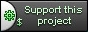# Difference "cppcms::base_view & cppcms::base_content" ver. 4 versus ver. 5

## Content:

 `` `## cppcms::base\_content` `This is a simple polymorphic class from which every cppcms content template should be derided. It allows dynamic casting of the content for the target application:` ` class base_content {` ` public:` ` virtual ~base_content() {};` ` };` `## cppcms::base\_view` `### Members` `- **`worker_thread &worker`** -- reference to the worker thread that called the rendering procedure.` `- **`ostream &cout`** -- reference to the output stream that output is rendered to.` `### How Derived Classes are created:` `This is the class that every view generated from the template system is derived from.` `Every derived class defines the important public member --- `content` --- the reference to the user defined content class.` `It also creates member functions for each implemented template. For example:` ` <% c++ #include "data.h" %>` ` <% namespace my_view %>` ` <% class message uses data::message %>` ` <% template render() %>` ` ` ` ` `

<% message %> World!

` ` ` ` ` ` <% end template %>` ` <% end class %>` ` <% end namespace %>` `The above template is rendered to C++ output:` ` #include "data.h" ` ` namespace my_view {` ` struct message :public cppcms::base_view` ` {` ` data::message &content;` ` cppcms::transtext::trans const *tr;` ` message(cppcms::base_view::settings _s,data::message &_content):` ` cppcms::base_view(_s),content(_content)` ` {` ` tr=_s.worker->domain_gettext("my_view");` ` };` ` virtual void render() {` ` cout<<"\n"` ` "\n"` ` " \n"` ` "

";` ` cout<\n"` ` " \n"` ` "\n"` ` "";` ` } // end of template render` ` }; // end of class message` ` } // end of namespace my_view` `### Member functions` `#### Default filter` ` template` ` string escape(T const &v)` `This is the default filter. It performs the rendering of the input parameter to text. This function is always called when the template parameter is substituted without filters, i.e.:` ` <% param %>` `is converted to` ` cout<` `- **`string intf(int val,string f)`** -- format integer number. It uses `boost::format` for this purpose. For example:` ` Decimal <% num %> is hexadecimal <% num | intf("%x"); %>` `- **`string strftime(std::tm const &t,string f)`** -- formats the output string using std::strftime call. For example:` ` <% date | strftime("%d/%m/%Y") %>` ` It has tho following "shortcuts" for showing the date only, the time or the time with seconds: ` ` string date(std::tm const &t);` ` string time(std::tm const &t);` ` string timesec(std::tm const &t);` `- **`string urlencode(string const &s);`** -- encode string for URL. For example:` ` ` `#### Other member functions` ` boost::format format(string const &f);` `Function that returns i\a `boost::format` object constructed with `f` and with disabled exceptions. In generally `boost::format` throws an exception in case of an incorrect format or parameters list --- this is not such a good behavior for displaying various data.` `It is used mostly by gettext and ngettext implementations. For example` ` <% ngt "We have one apple","We have %1% apples",n using n %>` `Is rendered to:` ` cout<gettext("We have one apple","We have %1% apples",n)) % escape(n);` `Thus it would not throw an exception in case of "one apple".` `This content is down.`

## Sidebar:

 `## Releated` `- [cppcms::application](/wikipp/en/page/ref_cppcms_application)` `This content is down.`

CppCMS is a web development framework for performance demanding applications.

## Support This Project